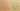AssignCompares

Misconception:

The = operator compares two values; e.g., 1 = 2 will evaluate to False, because 1 is not equal to 2.Incorrect

= compares two values

Correct

= assigns a value to a variable

CorrectionHere is what's right.

In Python, and in many other languages, the = operator does not compare values, but it assigns the value on its right to the variable on its left.

Thus, a = 1 will store a reference to the value 1 in the variable a.

This also means that 1 = 2 will cause a syntax error, because the left-hand-side of the assignment (1) does not represent a variable, which means that it’s not possible to store a value in it. The interpreter will report an error when it encounters expressions like 1 = 2.

OriginWhere could this misconception come from?

This misconception may stem from prior knowledge in mathematics, where = means equals (i.e., is a comparison operator).

SymptomsHow do you know your students might have this misconception?

Students may use (inadvertently or not) a single = operator in expressions used as conditions.

The most common place where the mistake happens is probably within if statements, such as:

if x = 1:
...

ValueHow can you build on this misconception?

In other programming languages (such as Pascal), the = operator indeed performs a comparison between its operands. In those languages, a different operator (e.g, := ) is used for an assignment.

Python